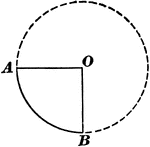### Sector of Circle

Illustration of a circle with a sector. "A sector of a circle is the space included between an arc and…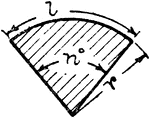### Sector Of Circle

Illustration of a sector of a circle. A sector is the space between an arc and two radii drawn to the…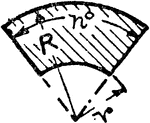### Sector Of A Hollow Circle

Illustration of a sector of a hollow circle. A sector is the space between an arc and two radii drawn…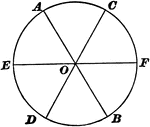### Sectors of Circle

Illustration of a circle with 6 equal sectors.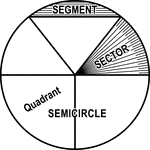### Circle with Segment, Semicircle, Sector, Quadrant, and Central Angle

Illustrations of a circle with segment, semicircle, sector, quadrant, and central angles drawn.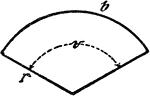### Circle Sector

An illustration showing a circle sector with radius r, center/central angle v, and length of circle…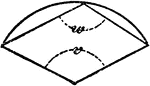### Circle Sector

An illustration showing a circle sector with center/central angle v and polygon angle w.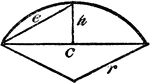### Circle Sector

An illustration showing a circle sector with height of segment h and radius r.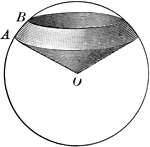### Spherical Sector

Illustration of a spherical sector.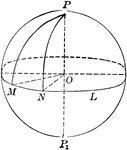### Sphere With Sectors and Arcs

Diagram of a sphere with sectors and arcs drawn.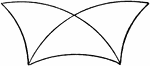### Superimposed Symmetrical Spherical Triangles

Diagram showing 2 superimposed symmetrical spherical triangles.### Symmetrical Spherical Triangles

Diagram showing 2 symmetrical spherical triangles.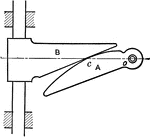### Logarithmic Spiral Sector

In this illustration, "the driven surface of the slide coincides with the tangent to the spiral, the…### Circle Inscribed In A Triangle

An illustration showing a circle with sectors D and E inscribed in a triangle with angles, A, B, and…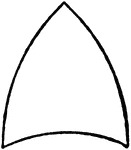### Spherical Triangle

Diagram showing a spherical triangle.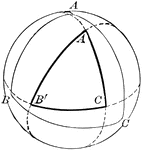### Spherical Triangle

Diagram showing a spherical triangle constructed by the intersection of polar arcs.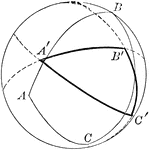### Spherical Triangle

Diagram showing a spherical triangle constructed by the intersection of polar arcs.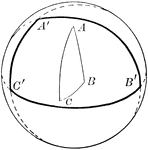### Spherical Triangle

Diagram showing a spherical triangle constructed by the intersection of polar arcs.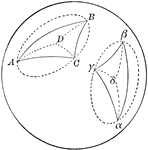### Two Symmetrical Spherical Triangles

Diagram showing a 2 symmetrical spherical triangles.